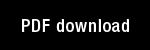On the variational systems of some nonlinear difference-differential equations

Author(s): Grossberg, S. |

Year: 1969

Citation: Journal of Differential Equations, 6, 544-577

Abstract: This paper studies the variational systems of two closely related systemsof nonlinear difference-differential equations which arise in prediction- andlearning-theoretical applications (, , [31). The first system is -IXxi(t)Nxm(t - T)Ymi(t), (1) M=1 yjk(t) = zjk(t) E zjm(t) - [ m-1 ]and zjk(t) = -uzjk(t) + Nxj(t - T) xk(t), (3)i, j, k = 1, 2,..., n, where n is any integer greater than 1, T is any nonnegativetime lag, and > 0. The second system differs from the first only in that (3)is replaced by 'Zj k(t) = -uzjk(t) + Pxj(t - T) xk(t), j # kand zjj(t) 0,j, k = 1, 2,..., n. Nonetheless, the qualitative behavior of (*) and (**) differdramatically as t --> co.(*) and (**) can be interpreted as cross-correlated flows over directedprobabilistic networks G(*) and G(**), respectively . Both G(*) and G(**)have n vertices V = {vi : i = 1, 2,..., n} and nz directed edgesE _ {ejk : j, k = 1, 2,..., n}.In G(*), each edge ejk is assigned the weight 9 (ejk) = 1/n. By contrast, inG(**) each edge ejk leading from a given vertex vi to a distinct vertex v7,, k =Aj,

Topics: Mathematical Foundations of Neural Networks, Models: Other,